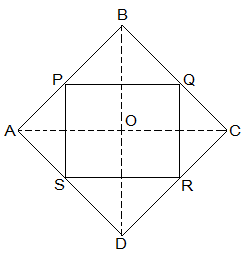Guru

# ABCD is a rhombus and P, Q, R and S are the mid-points of the sides AB, BC, CD and DA respectively. Show that the quadrilateral PQRS is a rectangle. Q.2

• 0

I want to know the best answer of the question from Quadrilaterals chapter of class 9th ncert math. The question from exercise 8.2 of math. Give me the easy way for solving this question of 2 ABCD is a rhombus and P, Q, R and S are the mid-points of the sides AB, BC, CD and DA respectively. Show that the quadrilateral PQRS is a rectangle.

Share

1.Given in the question,

ABCD is a rhombus and P, Q, R and S are the mid-points of the sides AB, BC, CD and DA respectively.

To Prove,

PQRS is a rectangle.

Construction,

Join AC and BD.

Proof:

In ΔDRS and ΔBPQ,

DS = BQ (Halves of the opposite sides of the rhombus)

∠SDR = ∠QBP (Opposite angles of the rhombus)

DR = BP (Halves of the opposite sides of the rhombus)

, ΔDRS ≅ ΔBPQ [SAS congruency]

RS = PQ [CPCT]———————- (i)

In ΔQCR and ΔSAP,

RC = PA (Halves of the opposite sides of the rhombus)

∠RCQ = ∠PAS (Opposite angles of the rhombus)

CQ = AS (Halves of the opposite sides of the rhombus)

, ΔQCR ≅ ΔSAP [SAS congruency]

RQ = SP [CPCT]———————- (ii)

Now,

In ΔCDB,

R and Q are the mid points of CD and BC respectively.

⇒ QR || BD

also,

P and S are the mid points of AD and AB respectively.

⇒ PS || BD

⇒ QR || PS

, PQRS is a parallelogram.

also, ∠PQR = 90°

Now,

In PQRS,

RS = PQ and RQ = SP from (i) and (ii)

∠Q = 90°

, PQRS is a rectangle.

• 0